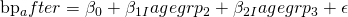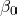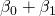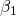# Why I think Stata’s old xi: prefix is still useful

A few versions ago Stata introduced a new facility for handling factor variables, which in many ways is superior to the older system, which was based on prefixing regression commands with xi:. But I actually think using this older xi: syntax can be useful in some situations, one of which is when trying to understand and learn about regression model specification.

To illustrate, let’s analyse one of Stata’s built in datasets:

sysuse bpwide.dta, clear


We will fit a linear regression model with the bp_after variable as outcome and the categorical variable agegrp as a predictor. Tabulating the agegrp variable we see that it takes three different values:

. tab agegrp

Age Group |      Freq.     Percent        Cum.
------------+-----------------------------------
30-45 |         40       33.33       33.33
46-59 |         40       33.33       66.67
60+ |         40       33.33      100.00
------------+-----------------------------------
Total |        120      100.00


To see how the variable is actually coded, we can add the nolabel option to tabulate:

. tab agegrp, nolabel

Age Group |      Freq.     Percent        Cum.
------------+-----------------------------------
1 |         40       33.33       33.33
2 |         40       33.33       66.67
3 |         40       33.33      100.00
------------+-----------------------------------
Total |        120      100.00


To fit a regression model for the bp_after variable with the agegrp variable as a categorical/factor predictor, using Stata’s newer factor variable notation we use:

. reg bp_after i.agegrp

Source |       SS       df       MS              Number of obs =     120
-------------+------------------------------           F(  2,   117) =   12.87
Model |  4312.86667     2  2156.43333           Prob > F      =  0.0000
Residual |   19606.725   117  167.578846           R-squared     =  0.1803
Total |  23919.5917   119  201.004972           Root MSE      =  12.945

------------------------------------------------------------------------------
bp_after |      Coef.   Std. Err.      t    P>|t|     [95% Conf. Interval]
-------------+----------------------------------------------------------------
agegrp |
46-59  |       6.45    2.89464     2.23   0.028     .7173166    12.18268
60+  |      14.65    2.89464     5.06   0.000     8.917317    20.38268
|
_cons |    144.325    2.04682    70.51   0.000     140.2714    148.3786
------------------------------------------------------------------------------


Here the estimated constant, 144.325, is the estimated mean in the lowest age group. The coefficient for the 46-59 group, 6.45, is the difference between the 46-59 group mean and the 30-45 group mean. Similarly, 14.65 is the difference in the mean of the outcome between the 60+ group and the 30-45 group.

In terms of understanding how the model is fitted however, the older xi: notation may I think be more useful, mainly because if we use the xi: notation Stata generates new variables into our dataset:

. xi: reg bp_after i.agegrp
i.agegrp          _Iagegrp_1-3        (naturally coded; _Iagegrp_1 omitted)

Source |       SS       df       MS              Number of obs =     120
-------------+------------------------------           F(  2,   117) =   12.87
Model |  4312.86667     2  2156.43333           Prob > F      =  0.0000
Residual |   19606.725   117  167.578846           R-squared     =  0.1803
Total |  23919.5917   119  201.004972           Root MSE      =  12.945

------------------------------------------------------------------------------
bp_after |      Coef.   Std. Err.      t    P>|t|     [95% Conf. Interval]
-------------+----------------------------------------------------------------
_Iagegrp_2 |       6.45    2.89464     2.23   0.028     .7173166    12.18268
_Iagegrp_3 |      14.65    2.89464     5.06   0.000     8.917317    20.38268
_cons |    144.325    2.04682    70.51   0.000     140.2714    148.3786
------------------------------------------------------------------------------


The estimates are of course the same as before, but the coefficient names are reported slightly differently, and the names correspond directly to the newly generated dummy/indicator variables in the dataset. If we list the value of the agegrp variable and the newly created dummy variables in three different observations (which have agegrp=1, agegrp=2 and agegrp=3), we see the following:

. list agegrp _Iagegrp_2 _Iagegrp_3 in 1

+------------------------------+
| agegrp   _Iageg~2   _Iageg~3 |
|------------------------------|
1. |  30-45          0          0 |
+------------------------------+

. list agegrp _Iagegrp_2 _Iagegrp_3 in 60

+------------------------------+
| agegrp   _Iageg~2   _Iageg~3 |
|------------------------------|
60. |  46-59          1          0 |
+------------------------------+

. list agegrp _Iagegrp_2 _Iagegrp_3 in 120

+------------------------------+
| agegrp   _Iageg~2   _Iageg~3 |
|------------------------------|
120. |    60+          0          1 |
+------------------------------+


Thus we see that the newly created variable _Iagegrp_2 takes the value 1 when agegrp=2, and 0 otherwise. Similarly _Iagegrp_3 equals 1 when agegrp=3, and 0 otherwise. Using these definitions, we can then express the regression equation as followsIn terms of understand what the regression model is assuming this approach makes crystal clear what is going on and why the coefficients mean what they do. When both dummy indicators are zero, the expected value of the outcome is equal to the intercept. When agegrp=2, _Iagegrp_2=1 and so the expected value of the outcome is. Thus we see that, the coefficient of _Iagegrp_2, corresponds to the difference between the mean of the outcome for agegrp=2 and for agegrp=1.

While Stata’s new factor notation, which doesn’t require xi: to be used as a prefix, I still find the xi: prefix useful, particularly when teaching, to help understand how the model equation is set up when one has categorical or factor predictors. Further, once you start including complex interactions into models, having a clear understanding of what the model equation is is particularly important.

This site uses Akismet to reduce spam. Learn how your comment data is processed.